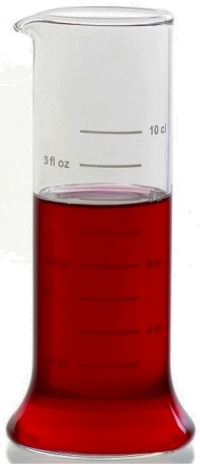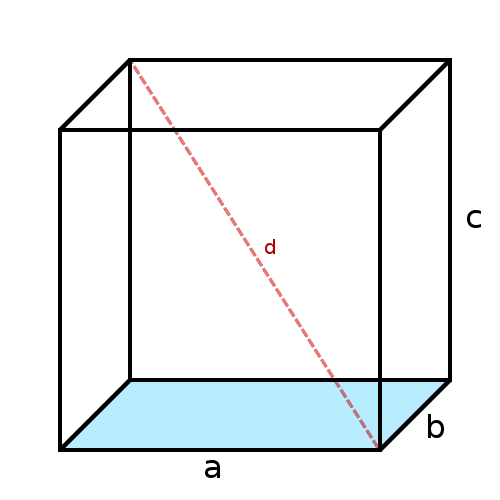## Volume conversionThis converter allows you to simultaneously convert a volume in several common units like cubic metre (base unit of volume in the International System of units - SI), litre (liter), gallon, liquid ounce, pint,barrel... Enter the volume in the second column of following array and the conversion will be performed automatically in all other units.

Common volume units :
 cubic metre m3 cubic centimetre cm3 litre l millilitre ml centilitre cl cubic foot ft3 cubic inch in3 gallon (USA) gallon (USA) liquid pint (USA) pint (USA) American liquid ounce fl oz (USA)

1 liquid ounce (fl oz) is equivalent to about 3 centilitres (cl)Volume unit less used :
 cubic millimetre mm3 decilitre dl decalitre dal hectolitre hl cubic kilometre km3 cubic mile mi3 cubic hectometre hm3 cubic decametre dam3 cubic decimetre (or litre) dm3 ou l tablespoon tbs tea spoon tsp barrel (for petrol)bbl imperial bushel (GB)bsh (GB) US bushel bsh (USA) cubic yard yd3 imperial gallon (GB) gallon (GB) imperial pint (GB) pint (GB) imperial liquid ounce (GB) fl oz (GB)See here conversion of heating wood storage volume

## Equivalence between the volume and the size of a cube

Compute the side or diagonal of a cube from its volume :volume in m3 side a in metres m diagonal d in metres m

## Equivalence between the volume and the radius of a sphere (globe, ball)

Compute the radius or the diameter of a globe (sphere) from its volume :volume in m3 radius r in metres m diametre in metres m surface in square metres m2